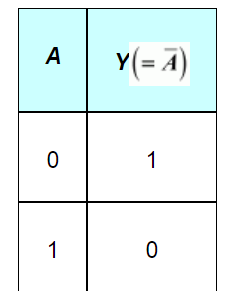Deepak Scored 45->99%ile with Bounce Back Crack Course. You can do it too!

# Write the truth table for a NAND gate connected as given in Fig. 14.45.

Question:

Write the truth table for a NAND gate connected as given in Fig. 14.45.Hence identify the exact logic operation carried out by this circuit.

Solution:

A acts as the two inputs of the NAND gate and Y is the output, as shown in the following figure.Hence, the output can be written as:

$Y=\overline{A \cdot A}=\bar{A}+\bar{A}=\bar{A}$    ...(1)

The truth table for equation (i) can be drawn as:This circuit functions as a NOT gate. The symbol for this logic circuit is shown as: